# NEITON watch hands green luminous fit NH35 NH36 ETA 2836 2824 Miyota 8205 8215 821A Mingzhu 2813 3804 movement watch hands

\$13.80

 color: Choose an optionNumber 01Number 02Number 03Number 04Number 05Number 06Number 07Number 08Number 09Number 10Number 11Number 12Number 13Number 14Number 15Number 16Number 17Number 18Number 19Number 20Number 21Number 22Number 23Number 24Number 25Number 26Number 27Number 28Number 29Number 30Number 31Number 32Number 33Number 34Number 35Number 36Number 37Number 38Number 39Number 40Number 41Number 42Number 43Number 44Number 45Number 46Number 47Number 48Number 49Number 50Number 51Number 52Number 53Number 54Number 55Number 56Number 57Number 58Number 59Number 60Number 61Number 62Number 63Number 64Number 65Number 66Number 67Number 68Number 69Number 70Number 71Number 72Number 73Number 74Number 75 Number 01 Number 02 Number 03 Number 04 Number 05 Number 06 Number 07 Number 08 Number 09 Number 10 Number 11 Number 12 Number 13 Number 14 Number 15 Number 16 Number 17 Number 18 Number 19 Number 20 Number 21 Number 22 Number 23 Number 24 Number 25 Number 26 Number 27 Number 28 Number 29 Number 30 Number 31 Number 32 Number 33 Number 34 Number 35 Number 36 Number 37 Number 38 Number 39 Number 40 Number 41 Number 42 Number 43 Number 44 Number 45 Number 46 Number 47 Number 48 Number 49 Number 50 Number 51 Number 52 Number 53 Number 54 Number 55 Number 56 Number 57 Number 58 Number 59 Number 60 Number 61 Number 62 Number 63 Number 64 Number 65 Number 66 Number 67 Number 68 Number 69 Number 70 Number 71 Number 72 Number 73 Number 74 Number 75 Number 01 Number 01 Number 02 Number 02 Number 03 Number 03 Number 04 Number 04 Number 05 Number 05 Number 06 Number 06 Number 07 Number 07 Number 08 Number 08 Number 09 Number 09 Number 10 Number 10 Number 11 Number 11 Number 12 Number 12 Number 13 Number 13 Number 14 Number 14 Number 15 Number 15 Number 16 Number 16 Number 17 Number 17 Number 18 Number 18 Number 19 Number 19 Number 20 Number 20 Number 21 Number 21 Number 22 Number 22 Number 23 Number 23 Number 24 Number 24 Number 25 Number 25 Number 26 Number 26 Number 27 Number 27 Number 28 Number 28 Number 29 Number 29 Number 30 Number 30 Number 31 Number 31 Number 32 Number 32 Number 33 Number 33 Number 34 Number 34 Number 35 Number 35 Number 36 Number 36 Number 37 Number 37 Number 38 Number 38 Number 39 Number 39 Number 40 Number 40 Number 41 Number 41 Number 42 Number 42 Number 43 Number 43 Number 44 Number 44 Number 45 Number 45 Number 46 Number 46 Number 47 Number 47 Number 48 Number 48 Number 49 Number 49 Number 50 Number 50 Number 51 Number 51 Number 52 Number 52 Number 53 Number 53 Number 54 Number 54 Number 55 Number 55 Number 56 Number 56 Number 57 Number 57 Number 58 Number 58 Number 59 Number 59 Number 60 Number 60 Number 61 Number 61 Number 62 Number 62 Number 63 Number 63 Number 64 Number 64 Number 65 Number 65 Number 66 Number 66 Number 67 Number 67 Number 68 Number 68 Number 69 Number 69 Number 70 Number 70 Number 71 Number 71 Number 72 Number 72 Number 73 Number 73 Number 74 Number 74 Number 75 Number 75 Clear
SKU: 1005002571450893 Category:
• Brand Name: Neiton
• Origin: Mainland China
• Material: Stainless steel
• Movement: fit ETA 2836 2824 NH35 NH36 Mingzhu 2813,3804 Miyota 82 series

Description

Luminous:Green luminous
Size:12.5mm x12mm x8mm (All measure from the center of hole to the end)
Movement:fit ETA 2836 2824 NH35 NH36 Mingzhu 2813,3804 Miyota 82 series movement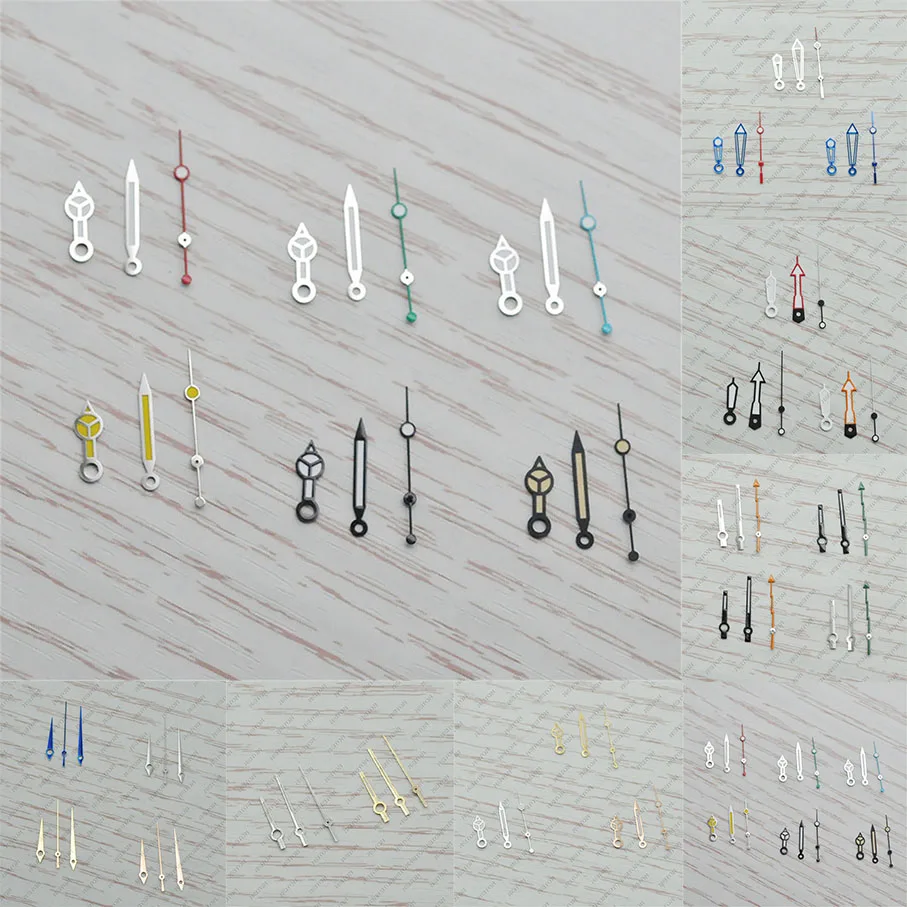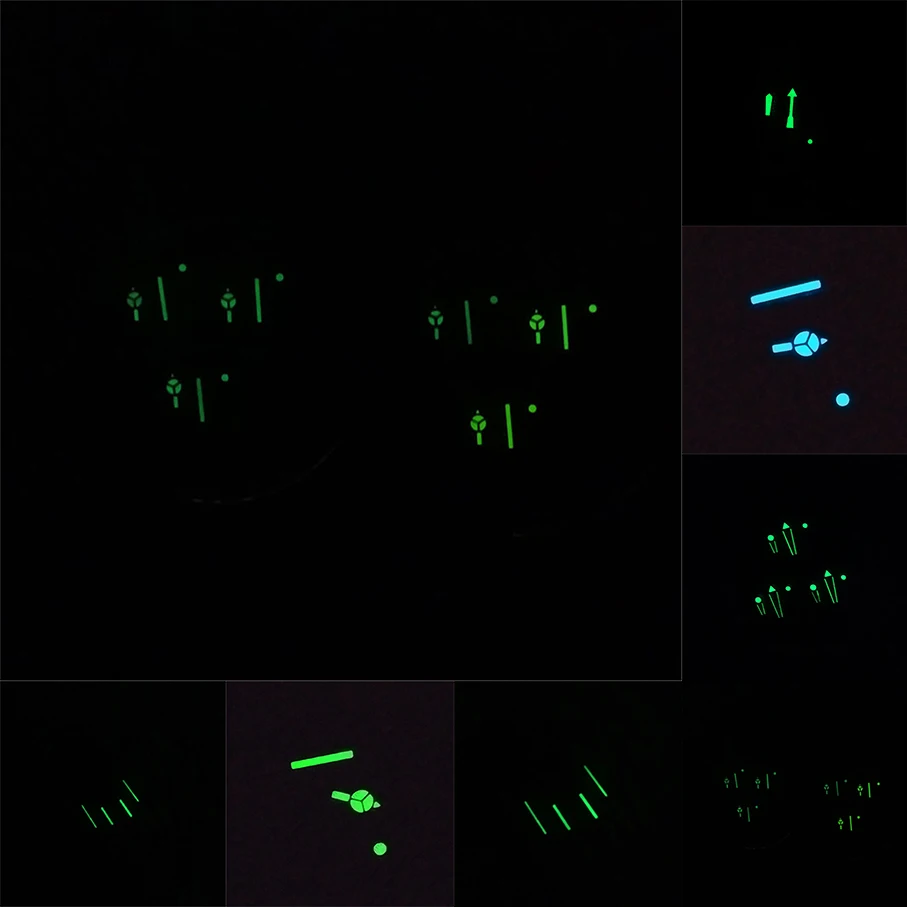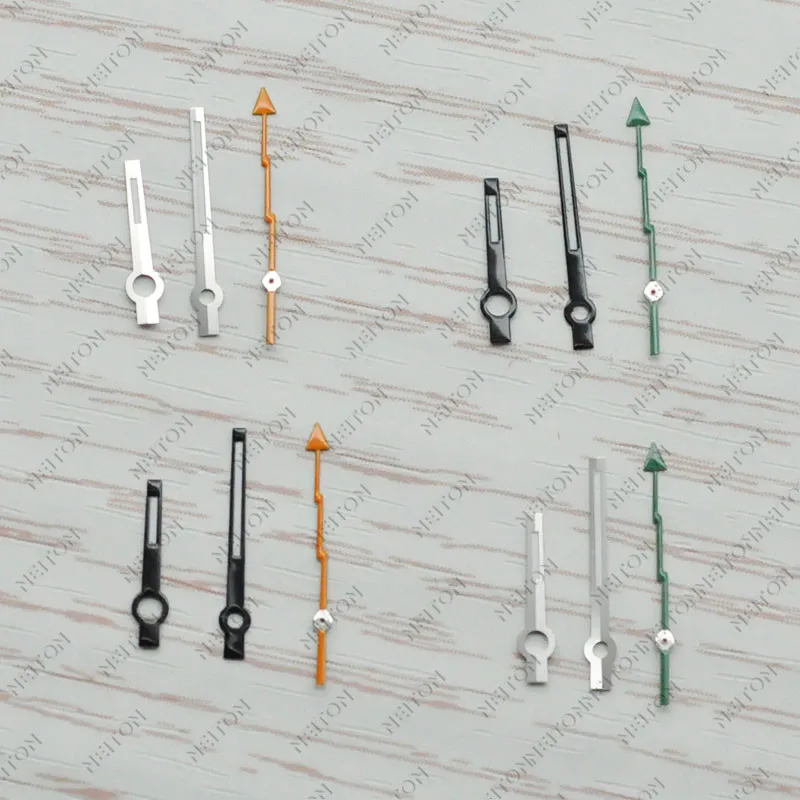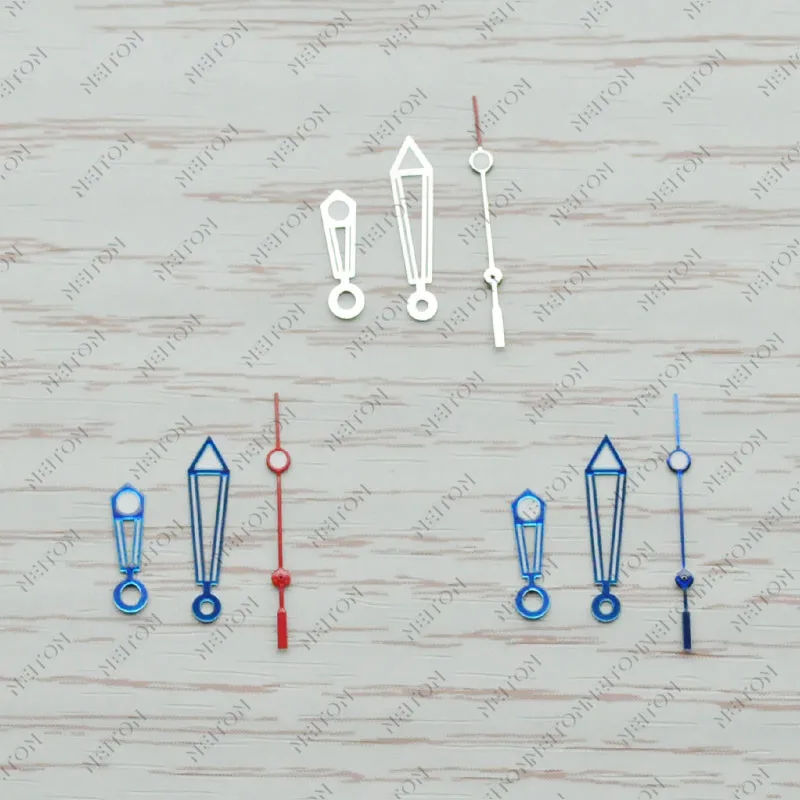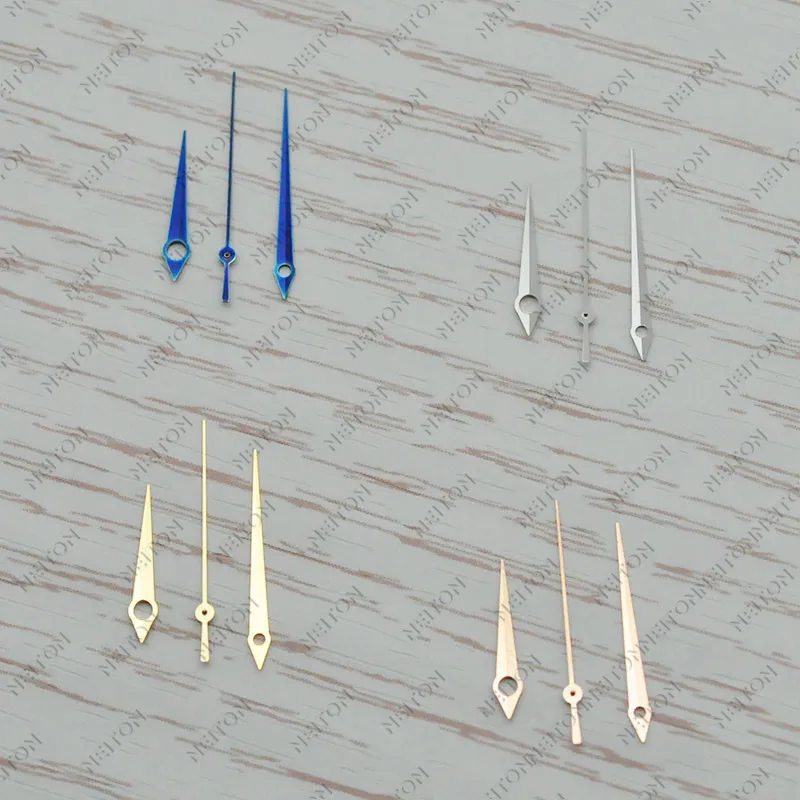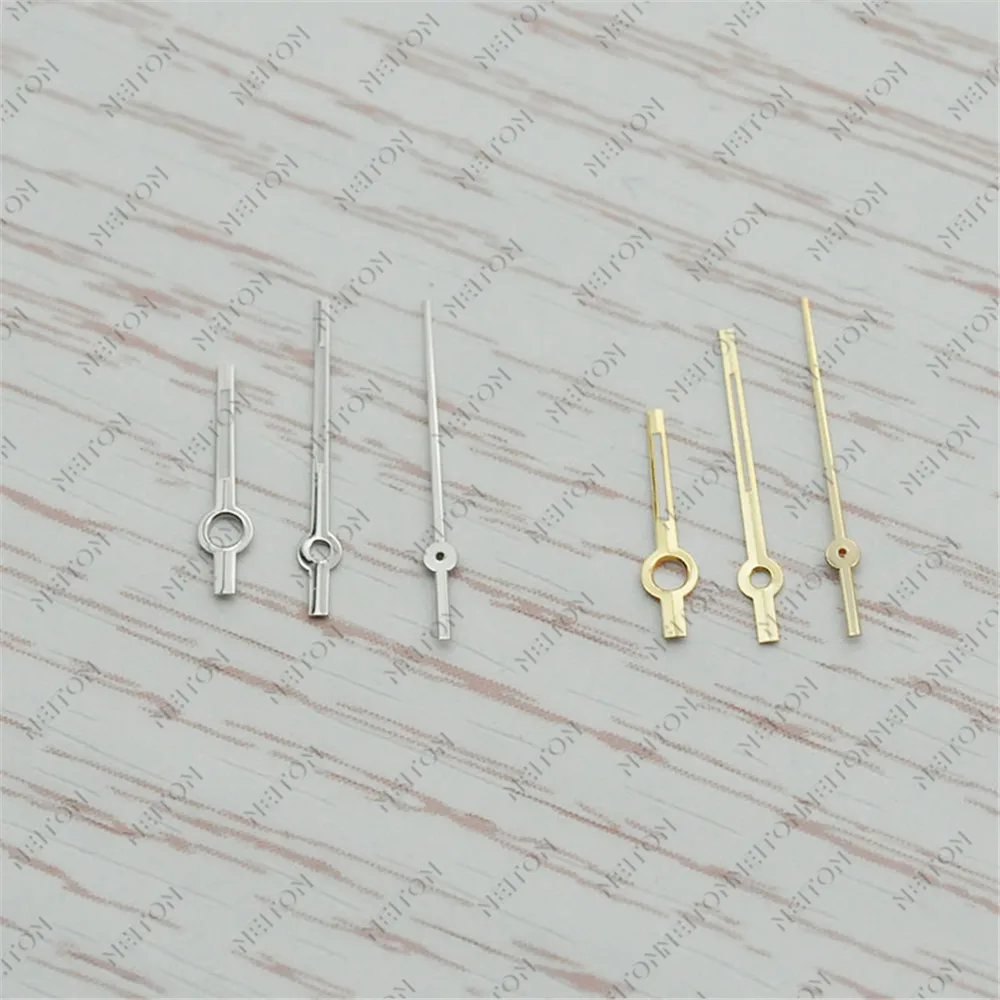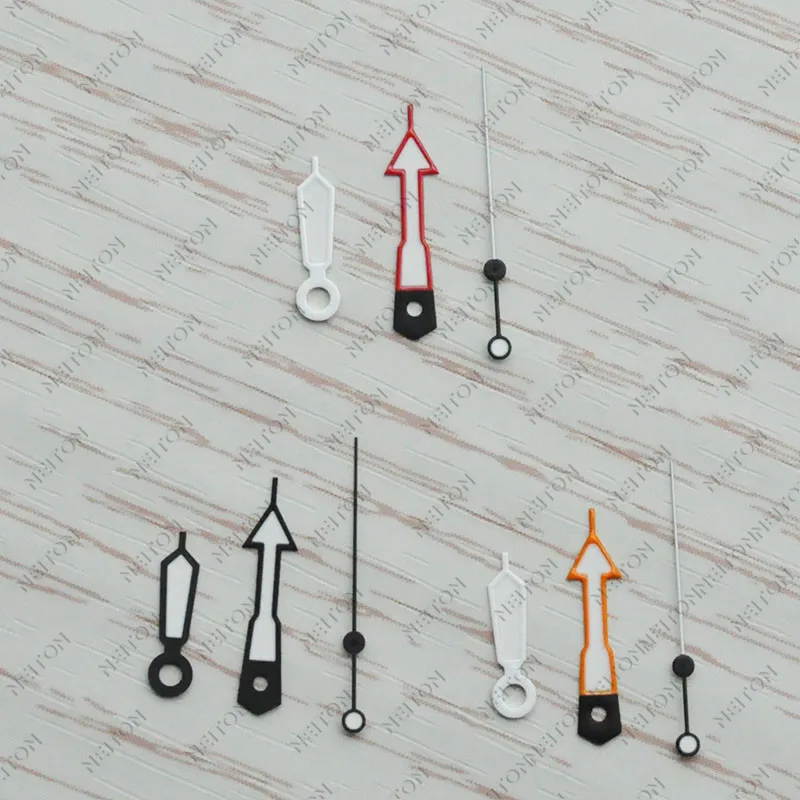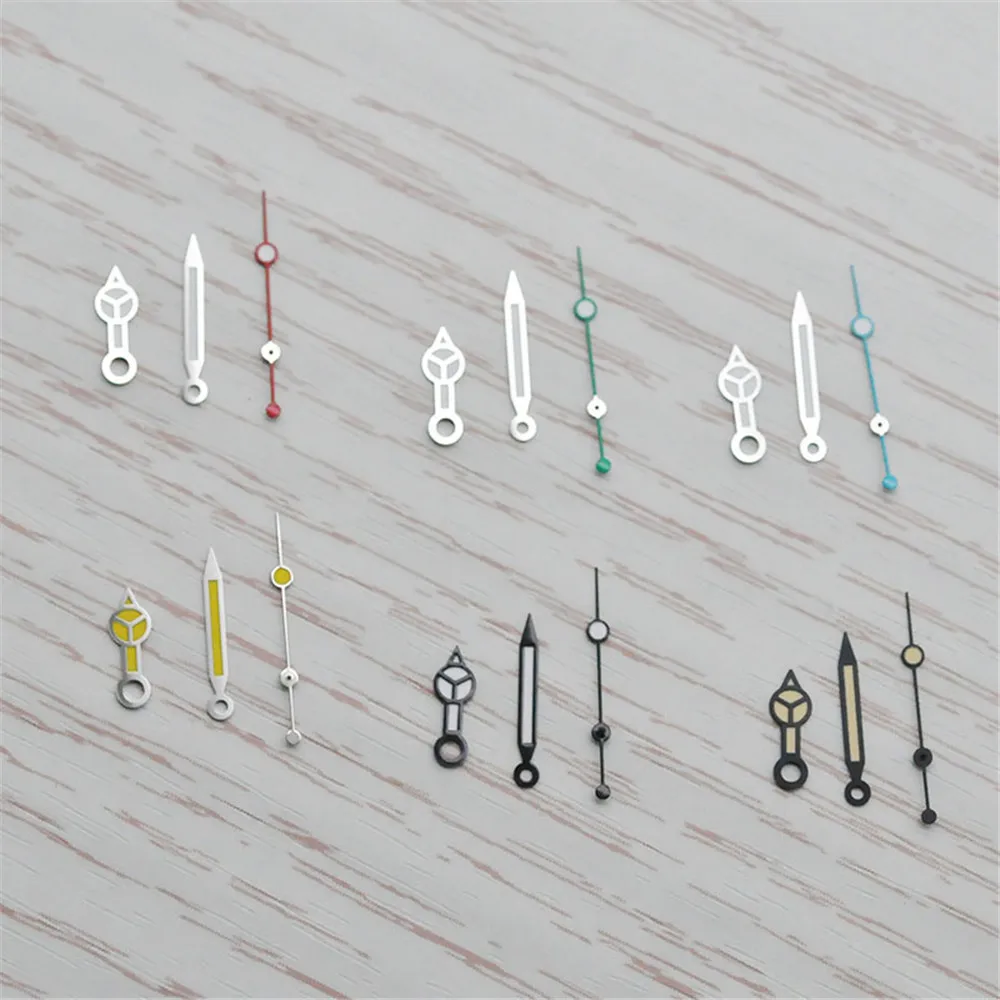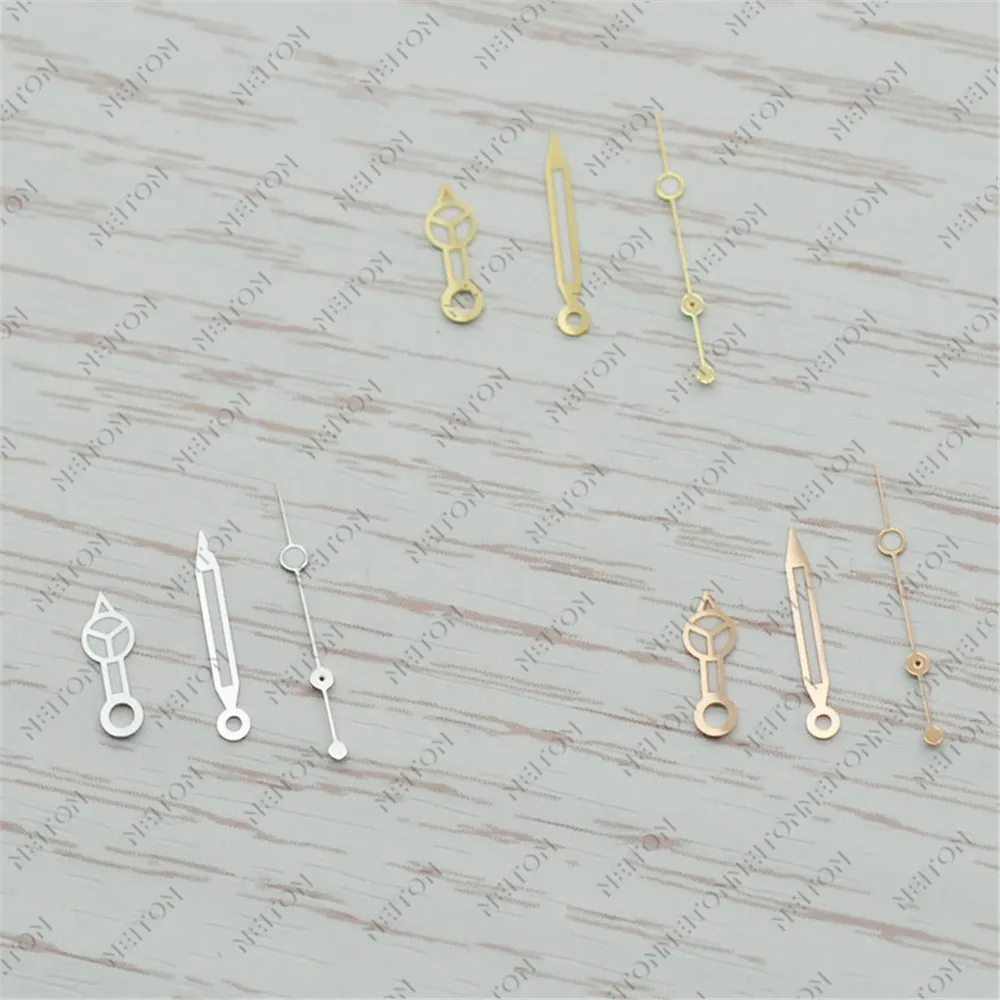## Reviews

There are no reviews yet.

## See It Styled On Instagram